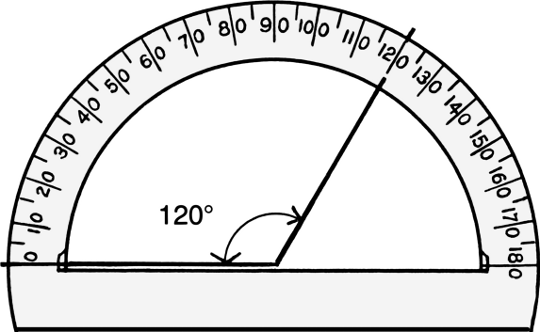# Definition of Degree (Angles)Degrees are one possible way of measuring angles.

We use the symbol ${}^\circ$ for degrees.

There are $360^\circ$ in a full rotation.

A straight angle has $180^\circ$, and a right angle is $90^\circ$.

We can use a protractor like the one in the picture to measure angles in degrees.

### Description

The aim of this dictionary is to provide definitions to common mathematical terms. Students learn a new math skill every week at school, sometimes just before they start a new skill, if they want to look at what a specific term means, this is where this dictionary will become handy and a go-to guide for a studen

### Audience

Year 1 to Year 12 students

### Learning Objectives

Learn common math terms starting with letter D

Author: Subject Coach
You must be logged in as Student to ask a Question.